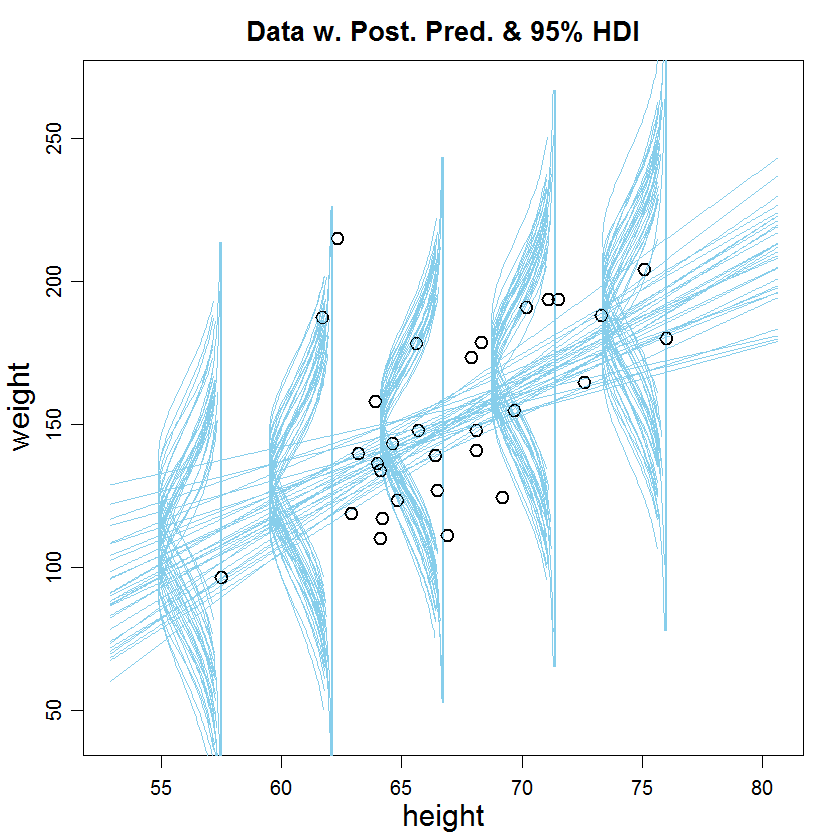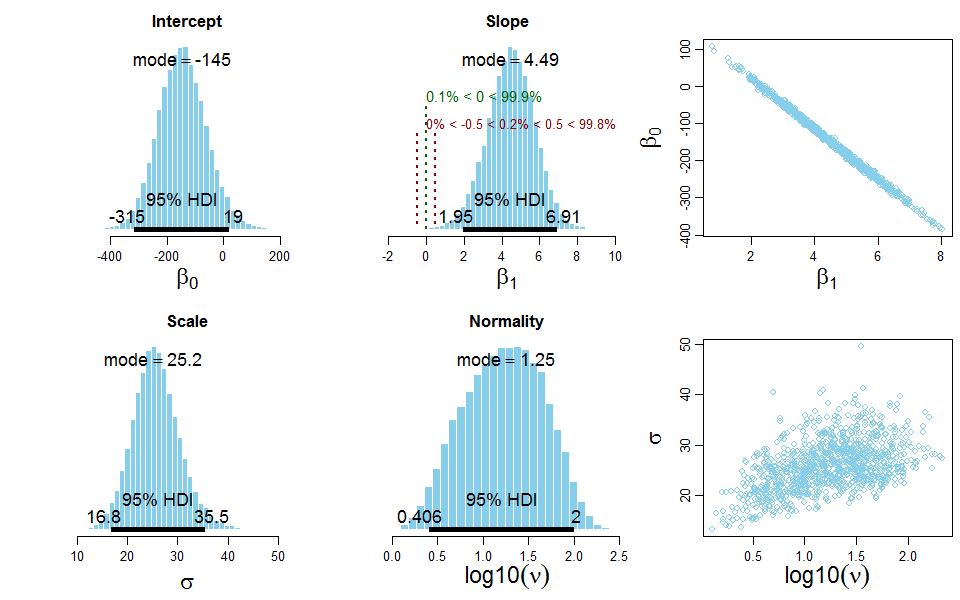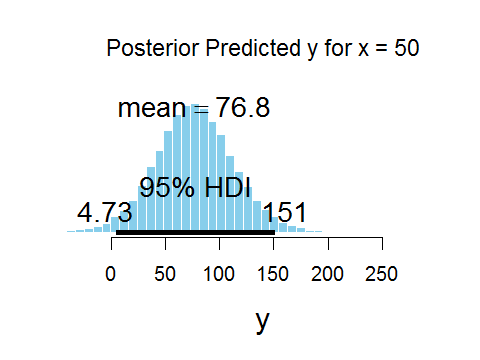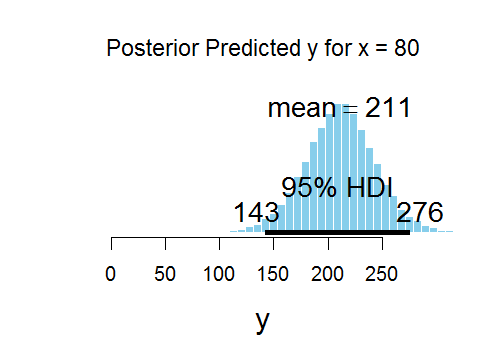## Saturday, October 10, 2015

### Posterior predicted distribution for linear regression in JAGS

A reader asked how to create posterior predicted distributions of data values, specifically in the case of linear regression. In other words, having done a simple linear regression analysis for some data, then, for a given probe value of x, what is the posterior distribution of predicted values for y? This topic is discussed in DBDA1E quite a lot (see, e.g., Section 16.1.3, p. 427, and the accompanying code on p. 441), but relatively little in DBDA2E. :-( This post shows an example of generating a posterior predictive distribution for simple linear regression in JAGS.

Update: A more recent post extends this to multiple linear regression.

The basic idea is as follows: At every step in the MCMC chain, use the parameter values at that step to randomly generate a simulated value of y from the model (for the probe value of x). Across the chain, the distribution of simulated y values is the posterior predictive distribution of y at x. There are two ways to program this process. Either (i) in R after JAGS has created the chain or (ii) in JAGS itself while it is creating the chain. In DBDA1E I preferred to do it in R but that was because I was using BUGS at the time and had encountered problems with BUGS. But an infelicity of doing it in R is that one has to re-write the entire model in R, outside of JAGS, and this can lead to errors in coding. Therefore this post shows an example of doing it in JAGS.

I modified the programs for simple linear regression that accompany DBDA2E: Jags-Ymet-Xmet-Mrobust.R and Jags-Ymet-Xmet-Mrobust-Example.R, and called them Jags-Ymet-Xmet-MrobustPredict.R and Jags-Ymet-Xmet-MrobustPredict-Example.R. I created a new argument, xProbe, that specifies the desired probe values of x in the genMCMC function. The changes in the the genMCMC are highlighted below:

genMCMC = function( data , xName="x" , yName="y" , xProbe=NULL ,
numSavedSteps=50000 , saveName=NULL ) {
require(rjags)
#-----------------------------------------------------------------------------
# THE DATA.
y = data[,yName]
x = data[,xName]
# Do some checking that data make sense:
if ( any( !is.finite(y) ) ) { stop("All y values must be finite.") }
if ( any( !is.finite(x) ) ) { stop("All x values must be finite.") }
if ( !is.null(xProbe) ) { # check that the xProbe values make sense
if ( any( !is.finite(xProbe) ) ) {
stop("All xProbe values must be finite.") }
} else { # fill in placeholder so JAGS doesn't balk
xProbe = quantile(x,probs=c(0.0,0.5,1.0))
}
#Ntotal = length(y)
# Specify the data in a list, for later shipment to JAGS:
dataList = list(
x = x ,
y = y ,
xP = xProbe

)
#-----------------------------------------------------------------------------
# THE MODEL.
modelString = "
# Standardize the data:
data {
Ntotal <- length(y)
xm <- mean(x)
ym <- mean(y)
xsd <- sd(x)
ysd <- sd(y)
for ( i in 1:length(y) ) {
zx[i] <- ( x[i] - xm ) / xsd
zy[i] <- ( y[i] - ym ) / ysd
}
Nprobe <- length(xP)
for ( j in 1:length(xP) ) { # standardize the xProbe values too!
zxP[j] <- ( xP[j] - xm ) / xsd
}
}
# Specify the model for standardized data:
model {
for ( i in 1:Ntotal ) {
zy[i] ~ dt( zbeta0 + zbeta1 * zx[i] , 1/zsigma^2 , nu )
}
# Priors vague on standardized scale:
zbeta0 ~ dnorm( 0 , 1/(10)^2 )
zbeta1 ~ dnorm( 0 , 1/(10)^2 )
zsigma ~ dunif( 1.0E-3 , 1.0E+3 )
nu <- nuMinusOne+1
nuMinusOne ~ dexp(1/29.0)
# Transform to original scale:
beta1 <- zbeta1 * ysd / xsd
beta0 <- zbeta0 * ysd  + ym - zbeta1 * xm * ysd / xsd
sigma <- zsigma * ysd
# Predicted y values at xProbe values:
for ( i in 1:Nprobe ) {
zyP[i] ~ dt( zbeta0 + zbeta1 * zxP[i] , 1/zsigma^2 , nu )
yP[i] <- zyP[i] * ysd + ym  # transform to original scale
}
}
" # close quote for modelString
# Write out modelString to a text file
writeLines( modelString , con="TEMPmodel.txt" )
#-----------------------------------------------------------------------------
# INTIALIZE THE CHAINS.
# Let JAGS do it...
#-----------------------------------------------------------------------------
# RUN THE CHAINS
parameters = c( "beta0" ,  "beta1" ,  "sigma",
"zbeta0" , "zbeta1" , "zsigma", "nu" , "xP" , "yP" )

The rest of the genMCMC function is unchanged. All we have to do after that is graph the predicted values. Here's some code to do the graphs:

# Plot posterior predicted y at x probe:
# Convert coda object to matrix:

mcmcMat = as.matrix(mcmcCoda)
# Find the xProbe and predicted y columns:
xPcols = grep( "xP" , colnames(mcmcMat) , value=FALSE )
yPcols = grep( "yP" , colnames(mcmcMat) , value=FALSE )

# Find the extreme predicted values for graph axis limits:
xLim = quantile( mcmcMat[,yPcols] , probs=c(0.005,0.995) )

# Make the plots of the posterior predicted values:
for ( i in 1:length(xPcols) ) {
openGraph(width=4,height=3)
plotPost( mcmcMat[,yPcols[i]] , xlab="y" , xlim=xLim , cenTend="mean" ,
main=bquote( "Posterior Predicted y for x = "
* .(mcmcMat[1,xPcols[i]]) )  )
}

Here's an example of the graphical output. First, the data with a smattering of credible regression lines:Now, the posterior distribution of the parameters:Finally, the distributions of posterior predicted y for two different probe values of x:Complete code for this example is appended below:

Jags-Ymet-Xmet-MrobustPredict.R

# Jags-Ymet-Xmet-MrobustPredict.R
# Accompanies the book:
#   Kruschke, J. K. (2015). Doing Bayesian Data Analysis:
#   A Tutorial with R, JAGS, and Stan 2nd Edition. Academic Press / Elsevier.

source("DBDA2E-utilities.R")

#===============================================================================

genMCMC = function( data , xName="x" , yName="y" , xProbe=NULL ,
numSavedSteps=50000 , saveName=NULL ) {
require(rjags)
#-----------------------------------------------------------------------------
# THE DATA.
y = data[,yName]
x = data[,xName]
# Do some checking that data make sense:
if ( any( !is.finite(y) ) ) { stop("All y values must be finite.") }
if ( any( !is.finite(x) ) ) { stop("All x values must be finite.") }
if ( !is.null(xProbe) ) {
if ( any( !is.finite(xProbe) ) ) {
stop("All xProbe values must be finite.") }
} else { # fill in placeholder so JAGS doesn't balk
xProbe = quantile(x,probs=c(0.0,0.5,1.0))
}
#Ntotal = length(y)
# Specify the data in a list, for later shipment to JAGS:
dataList = list(
x = x ,
y = y ,
xP = xProbe
)
#-----------------------------------------------------------------------------
# THE MODEL.
modelString = "
# Standardize the data:
data {
Ntotal <- length(y)
xm <- mean(x)
ym <- mean(y)
xsd <- sd(x)
ysd <- sd(y)
for ( i in 1:length(y) ) {
zx[i] <- ( x[i] - xm ) / xsd
zy[i] <- ( y[i] - ym ) / ysd
}
Nprobe <- length(xP)
for ( j in 1:length(xP) ) {
zxP[j] <- ( xP[j] - xm ) / xsd
}
}
# Specify the model for standardized data:
model {
for ( i in 1:Ntotal ) {
zy[i] ~ dt( zbeta0 + zbeta1 * zx[i] , 1/zsigma^2 , nu )
}
# Priors vague on standardized scale:
zbeta0 ~ dnorm( 0 , 1/(10)^2 )
zbeta1 ~ dnorm( 0 , 1/(10)^2 )
zsigma ~ dunif( 1.0E-3 , 1.0E+3 )
nu <- nuMinusOne+1
nuMinusOne ~ dexp(1/29.0)
# Transform to original scale:
beta1 <- zbeta1 * ysd / xsd
beta0 <- zbeta0 * ysd  + ym - zbeta1 * xm * ysd / xsd
sigma <- zsigma * ysd
# Predicted y values as xProbe:
for ( i in 1:Nprobe ) {
zyP[i] ~ dt( zbeta0 + zbeta1 * zxP[i] , 1/zsigma^2 , nu )
yP[i] <- zyP[i] * ysd + ym
}
}
" # close quote for modelString
# Write out modelString to a text file
writeLines( modelString , con="TEMPmodel.txt" )
#-----------------------------------------------------------------------------
# INTIALIZE THE CHAINS.
# Let JAGS do it...
#-----------------------------------------------------------------------------
# RUN THE CHAINS
parameters = c( "beta0" ,  "beta1" ,  "sigma",
"zbeta0" , "zbeta1" , "zsigma", "nu" , "xP" , "yP" )
adaptSteps = 500  # Number of steps to "tune" the samplers
burnInSteps = 1000
nChains = 4
thinSteps = 1
nIter = ceiling( ( numSavedSteps * thinSteps ) / nChains )
# Create, initialize, and adapt the model:
jagsModel = jags.model( "TEMPmodel.txt" , data=dataList , #inits=initsList ,
# Burn-in:
cat( "Burning in the MCMC chain...\n" )
update( jagsModel , n.iter=burnInSteps )
# The saved MCMC chain:
cat( "Sampling final MCMC chain...\n" )
codaSamples = coda.samples( jagsModel , variable.names=parameters ,
n.iter=nIter , thin=thinSteps )
# resulting codaSamples object has these indices:
#   codaSamples[[ chainIdx ]][ stepIdx , paramIdx ]
if ( !is.null(saveName) ) {
save( codaSamples , file=paste(saveName,"Mcmc.Rdata",sep="") )
}
return( codaSamples )
} # end function

#===============================================================================

smryMCMC = function(  codaSamples ,
compValBeta0=NULL , ropeBeta0=NULL ,
compValBeta1=NULL , ropeBeta1=NULL ,
compValSigma=NULL , ropeSigma=NULL ,
saveName=NULL ) {
summaryInfo = NULL
mcmcMat = as.matrix(codaSamples,chains=TRUE)
summaryInfo = rbind( summaryInfo ,
"beta0" = summarizePost( mcmcMat[,"beta0"] ,
compVal=compValBeta0 ,
ROPE=ropeBeta0 ) )
summaryInfo = rbind( summaryInfo ,
"beta1" = summarizePost( mcmcMat[,"beta1"] ,
compVal=compValBeta1 ,
ROPE=ropeBeta1 ) )
summaryInfo = rbind( summaryInfo ,
"sigma" = summarizePost( mcmcMat[,"sigma"] ,
compVal=compValSigma ,
ROPE=ropeSigma ) )
summaryInfo = rbind( summaryInfo ,
"nu" = summarizePost( mcmcMat[,"nu"] ,
compVal=NULL , ROPE=NULL ) )
summaryInfo = rbind( summaryInfo ,
"log10(nu)" = summarizePost( log10(mcmcMat[,"nu"]) ,
compVal=NULL , ROPE=NULL ) )
if ( !is.null(saveName) ) {
write.csv( summaryInfo , file=paste(saveName,"SummaryInfo.csv",sep="") )
}
return( summaryInfo )
}

#===============================================================================

plotMCMC = function( codaSamples , data , xName="x" , yName="y" ,
compValBeta0=NULL , ropeBeta0=NULL ,
compValBeta1=NULL , ropeBeta1=NULL ,
compValSigma=NULL , ropeSigma=NULL ,
showCurve=FALSE ,  pairsPlot=FALSE ,
saveName=NULL , saveType="jpg" ) {
# showCurve is TRUE or FALSE and indicates whether the posterior should
#   be displayed as a histogram (by default) or by an approximate curve.
# pairsPlot is TRUE or FALSE and indicates whether scatterplots of pairs
#   of parameters should be displayed.
#-----------------------------------------------------------------------------
y = data[,yName]
x = data[,xName]
mcmcMat = as.matrix(codaSamples,chains=TRUE)
chainLength = NROW( mcmcMat )
zbeta0 = mcmcMat[,"zbeta0"]
zbeta1 = mcmcMat[,"zbeta1"]
zsigma = mcmcMat[,"zsigma"]
beta0 = mcmcMat[,"beta0"]
beta1 = mcmcMat[,"beta1"]
sigma = mcmcMat[,"sigma"]
nu = mcmcMat[,"nu"]
log10nu = log10(nu)
#-----------------------------------------------------------------------------
if ( pairsPlot ) {
# Plot the parameters pairwise, to see correlations:
openGraph()
nPtToPlot = 1000
plotIdx = floor(seq(1,chainLength,by=chainLength/nPtToPlot))
panel.cor = function(x, y, digits=2, prefix="", cex.cor, ...) {
usr = par("usr"); on.exit(par(usr))
par(usr = c(0, 1, 0, 1))
r = (cor(x, y))
txt = format(c(r, 0.123456789), digits=digits)
txt = paste(prefix, txt, sep="")
if(missing(cex.cor)) cex.cor <- 0.8/strwidth(txt)
text(0.5, 0.5, txt, cex=1.25 ) # was cex=cex.cor*r
}
pairs( cbind( beta0 , beta1 , sigma , log10nu )[plotIdx,] ,
labels=c( expression(beta) , expression(beta) ,
expression(sigma) ,  expression(log10(nu)) ) ,
lower.panel=panel.cor , col="skyblue" )
if ( !is.null(saveName) ) {
saveGraph( file=paste(saveName,"PostPairs",sep=""), type=saveType)
}
}
#-----------------------------------------------------------------------------
# Marginal histograms:
# Set up window and layout:
nPtToPlot = 1000
plotIdx = floor(seq(1,chainLength,by=chainLength/nPtToPlot))
openGraph(width=8,height=5)
layout( matrix( 1:6 , nrow=2, byrow=TRUE ) )
par( mar=c(4,4,2.5,0.5) , mgp=c(2.5,0.7,0) )
histInfo = plotPost( beta0 , cex.lab = 1.75 , showCurve=showCurve ,
compVal=compValBeta0 , ROPE=ropeBeta0 ,
xlab=bquote(beta) , main=paste("Intercept") )
histInfo = plotPost( beta1 , cex.lab = 1.75 , showCurve=showCurve ,
compVal=compValBeta1 , ROPE=ropeBeta1 ,
xlab=bquote(beta) , main=paste("Slope") )
plot( beta1[plotIdx] , beta0[plotIdx] ,
xlab=bquote(beta) , ylab=bquote(beta) ,
col="skyblue" , cex.lab = 1.75 )
histInfo = plotPost( sigma , cex.lab = 1.75 , showCurve=showCurve ,
compVal=compValSigma , ROPE=ropeSigma ,
xlab=bquote(sigma) , main=paste("Scale") )
histInfo = plotPost( log10nu , cex.lab = 1.75 , showCurve=showCurve ,
compVal=NULL , ROPE=NULL ,
xlab=bquote(log10(nu)) , main=paste("Normality") )
plot( log10nu[plotIdx] , sigma[plotIdx] ,
xlab=bquote(log10(nu)) ,ylab=bquote(sigma) ,
col="skyblue" , cex.lab = 1.75 )
if ( !is.null(saveName) ) {
saveGraph( file=paste(saveName,"PostMarg",sep=""), type=saveType)
}
#-----------------------------------------------------------------------------
# Data with superimposed regression lines and noise distributions:
openGraph()
par( mar=c(3,3,2,1)+0.5 , mgp=c(2.1,0.8,0) )
# Plot data values:
postPredHDImass = 0.95
xRang = max(x)-min(x)
yRang = max(y)-min(y)
xLimMult = 0.25
yLimMult = 0.45
xLim= c( min(x)-xLimMult*xRang , max(x)+xLimMult*xRang )
yLim= c( min(y)-yLimMult*yRang , max(y)+yLimMult*yRang )
plot( x , y , cex=1.5 , lwd=2 , col="black" , xlim=xLim , ylim=yLim ,
xlab=xName , ylab=yName , cex.lab=1.5 ,
main=paste( "Data w. Post. Pred. & ",postPredHDImass*100,"% HDI" ,sep="") ,
cex.main=1.33  )
# Superimpose a smattering of believable regression lines:
nPredCurves=30
xComb = seq(xLim,xLim,length=501)
for ( i in floor(seq(from=1,to=chainLength,length=nPredCurves)) ) {
lines( xComb , beta0[i] + beta1[i]*xComb , col="skyblue" )
}
# Superimpose some vertical distributions to indicate spread:
#source("HDIofICDF.R")
nSlice = 5
curveXpos = seq(min(x),max(x),length=nSlice)
curveWidth = (max(x)-min(x))/(nSlice+2)
for ( i in floor(seq(from=1,to=chainLength,length=nPredCurves)) ) {
for ( j in 1:length(curveXpos) ) {
yHDI = HDIofICDF( qt , credMass=postPredHDImass , df=nu[i] )
yComb = seq(yHDI,yHDI,length=75)
xVals = dt( yComb , df=nu[i] )
xVals = curveWidth * xVals / dt(0,df=nu[i])
yPred = beta0[i] + beta1[i]*curveXpos[j]
yComb = yComb*sigma[i] + yPred
lines( curveXpos[j] - xVals , yComb , col="skyblue" )
lines( curveXpos[j] - 0*xVals , yComb , col="skyblue" , lwd=2 )
}
}
# replot the data, in case they are obscured by lines:
points( x , y , cex=1.5 )
if ( !is.null(saveName) ) {
saveGraph( file=paste(saveName,"PostPred",sep=""), type=saveType)
}
# if you want to show the y intercept, set this to TRUE:
showIntercept=TRUE
if ( showIntercept ) {
openGraph()
par( mar=c(3,3,2,1)+0.5 , mgp=c(2.1,0.8,0) )
# Plot data values:
xRang = max(x)-min(x)
yRang = max(y)-min(y)
xLimMult = 0.25
yLimMult = 0.45
xLim= c( min(x)-xLimMult*xRang , max(x)+xLimMult*xRang )
xLim = c(0,max(xLim))
yLim= c( min(y)-yLimMult*yRang , max(y)+yLimMult*yRang )
nPredCurves=30
pltIdx = floor(seq(from=1,to=chainLength,length=nPredCurves))
intRange = range( beta0[pltIdx] )
yLim = range( c(yLim,intRange) )
postPredHDImass = 0.95
plot( x , y , cex=1.5 , lwd=2 , col="black" , xlim=xLim , ylim=yLim ,
xlab=xName , ylab=yName , cex.lab=1.5 ,
main=paste( "Data w. Post. Pred. & ",postPredHDImass*100,"% HDI" ,sep="") ,
cex.main=1.33  )
abline(v=0,lty="dashed")
# Superimpose a smattering of believable regression lines:
xComb = seq(xLim,xLim,length=501)
for ( i in pltIdx  ) {
lines( xComb , beta0[i] + beta1[i]*xComb , col="skyblue" )
}
# Superimpose some vertical distributions to indicate spread:
#source("HDIofICDF.R")
nSlice = 5
curveXpos = seq(min(x),max(x),length=nSlice)
curveWidth = (max(x)-min(x))/(nSlice+2)
for ( i in floor(seq(from=1,to=chainLength,length=nPredCurves)) ) {
for ( j in 1:length(curveXpos) ) {
yHDI = HDIofICDF( qt , credMass=postPredHDImass , df=nu[i] )
yComb = seq(yHDI,yHDI,length=75)
xVals = dt( yComb , df=nu[i] )
xVals = curveWidth * xVals / dt(0,df=nu[i])
yPred = beta0[i] + beta1[i]*curveXpos[j]
yComb = yComb*sigma[i] + yPred
lines( curveXpos[j] - xVals , yComb , col="skyblue" )
lines( curveXpos[j] - 0*xVals , yComb , col="skyblue" , lwd=2 )
}
}
# replot the data, in case they are obscured by lines:
points( x , y , cex=1.5 )
if ( !is.null(saveName) ) {
saveGraph( file=paste(saveName,"PostPredYint",sep=""), type=saveType)
}
}
}

#===============================================================================

Jags-Ymet-Xmet-MrobustPredict-Example.R.

# Example for Jags-Ymet-Xmet-MrobustPredict.R
#-------------------------------------------------------------------------------
# Optional generic preliminaries:
graphics.off() # This closes all of R's graphics windows.
rm(list=ls())  # Careful! This clears all of R's memory!
#-------------------------------------------------------------------------------
# Load data file and specity column names of x (predictor) and y (predicted):
xName = "height" ; yName = "weight"
#xProbe=NULL
xProbe=seq(50,80,10)
fileNameRoot = "HtWtData30-Jags-"
#............................................................................
# Load data file and specity column names of x (predictor) and y (predicted):
# myData = read.csv( file="HtWtData300.csv" )
# xName = "height" ; yName = "weight"
# fileNameRoot = "HtWtData300-Jags-"
#............................................................................
graphFileType = "png"
#-------------------------------------------------------------------------------
# Load the relevant model into R's working memory:
source("Jags-Ymet-Xmet-MrobustPredict.R")
#-------------------------------------------------------------------------------
# Generate the MCMC chain:
#startTime = proc.time()
mcmcCoda = genMCMC( data=myData , xName=xName , yName=yName , xProbe=xProbe ,
numSavedSteps=50000 , saveName=fileNameRoot )
#stopTime = proc.time()
#duration = stopTime - startTime
#show(duration)

# #-------------------------------------------------------------------------------
# # Display diagnostics of chain, for specified parameters:
# parameterNames = varnames(mcmcCoda) # get all parameter names
# for ( parName in parameterNames ) {
#   diagMCMC( codaObject=mcmcCoda , parName=parName ,
#             saveName=fileNameRoot , saveType=graphFileType )
# }
# #-------------------------------------------------------------------------------
# # Get summary statistics of chain:
# summaryInfo = smryMCMC( mcmcCoda ,
#                         compValBeta1=0.0 , ropeBeta1=c(-0.5,0.5) ,
#                         saveName=fileNameRoot )
# show(summaryInfo)

# Display posterior information:
plotMCMC( mcmcCoda , data=myData , xName=xName , yName=yName ,
compValBeta1=0.0 , ropeBeta1=c(-0.5,0.5) ,
pairsPlot=TRUE , showCurve=FALSE ,
saveName=fileNameRoot , saveType=graphFileType )
#-------------------------------------------------------------------------------
# Plot posterior predicted y at x probe:
mcmcMat = as.matrix(mcmcCoda)
xPcols = grep( "xP" , colnames(mcmcMat) , value=FALSE )
yPcols = grep( "yP" , colnames(mcmcMat) , value=FALSE )
xLim = quantile( mcmcMat[,yPcols] , probs=c(0.005,0.995) )
for ( i in 1:length(xPcols) ) {
openGraph(width=4,height=3)
plotPost( mcmcMat[,yPcols[i]] , xlab="y" , xlim=xLim , cenTend="mean" ,
main=bquote( "Posterior Predicted y for x = "
* .(mcmcMat[1,xPcols[i]]) )  )
}
#-------------------------------------------------------------------------------

1.This is very helpful. Thank you.

I managed to do this in R using based on this program. Which looks to be from the first edition of the book.

The solution you post here seems better to me for the reason you state, but also because JAGS and R have different functions for sampling from a T distribution. My model in R looks something like this:

rt(1,df=mcmcMat[i,"nu"])*mcmcMat[i,"sigma"] + mcmcMat[i,"beta0"] + mcmcMat[i,"beta1"] * testX

This caused confusion for me for a while but eventually drove home the point that R and JAGS don't use the same functions.

Thank you again for posting this.

2.This looks to be very helpful! I'm teaching a course using your book this semester and know that this will save me hours of work trying to figure out how to do this.

Cheers!
Todd

3.One question.
In your example for x=50, the mean y=76.8, and for x=80, the mean y=211.
Would it be appropriate, at the same time we are doing the linear regression, to compare these two posterior predictive distributions using a contrast? The question would be whether the model predicts a credibly higher y outcome for x=80 compared to x=50.

4.Sure. After all, the posterior predictive distribution is merely a function of the posterior parameter distribution, just like a difference or parameters (e.g., μ1-μ2) or an effect size (e.g., μ/σ) is merely a function of parameters.

Also be careful if you're going to make a claim about "rejecting" a difference of zero. If you phrase it as a "hypothesis test" regarding the hypothesis that the difference is zero, then you are in the realm of a model comparison and should be considering Bayes factors and I'm not sure how that would work for predictive distributions. Instead if you phrase it in terms of intervals of the posterior predictive distribution then you should use some reasonable ROPE around the difference of zero.

5.Dear John, dear all,

I have been plotting posterior predictives using the fanplot package. For y values at different levels (not for regression but for a certain psychometric function in my case; but it could be applied here as well, I think) I densely generate predicted samples also for new levels in between the experimental levels. The higher the certainty, the more defined the course of the function gets. For low certainty, it will be very fuzzy. It's maybe not as accurate as plotting the little sideways gauss curves at some data points, but it's quite intuitive. Here is an example.

I'd be interested in your opinions regarding the usefulness of this visualization.

Best regards
Jan

6.7.Hello.
In your book, What is the meaning of "low-level parameters" ?

8.Skan: You don't provide any context for your question, but I suspect you are referring to hierarchical models. When displayed as a diagram, low-level parameters are usually lower in the diagram than high-level parameters. For example, parameters that describe individuals are lower-level than parameters that describe group tendencies. Mathematically, if the likelihood function can be factored like this:
p(D|a,b,c) = p(D|a) p(a|b) p(b|c) p(c)
then a is a lower-level parameter than b which is a lower-level parameter than c.

9.OK, thank you.
I was speaking about 9.3 Shrinkage in hierarchical models.

10.I've tried setting this for the multiple regression version, but the JAGS model won't compile.

The model below gives this error:
Error parsing model file:
syntax error on line 37 near "yP"

JAGS model syntax:
1 | data{
2 | ym <- mean(y)
3 | ysd <- sd(y)
4 | for ( i in 1:Ntotal ) {
5 | zy[i] <- ( y[i] - ym ) / ysd
6 | }
7 | for ( j in 1:Nx ) {
8 | xm[j] <- mean(x[,j])
9 | xsd[j] <- sd(x[,j])
10 | for ( i in 1:Ntotal ) {
11 | zx[i,j] <- ( x[i,j] - xm[j] ) / xsd[j]
12 | }
13 | }
14 | Nprobe <- length(xP)
15 | for ( j in 1:length(xP) ) {
16 | zxP[j] <- ( xP[j] - xm ) / xsd
17 | }
18 | }
19 | model{
20 | for ( i in 1:Ntotal ) {
21 | zy[i] ~ dt( zbeta0 + sum( zbeta[1:Nx] * zx[i,1:Nx] ) , 1/zsigma^2 , nu )
22 | }
23 | # Priors vague on standardized scale:
24 | zbeta0 ~ dnorm( 0 , 1/2^2 )
25 | for ( j in 1:Nx ) {
26 | zbeta[j] ~ dnorm( 0 , 1/2^2 )
27 | }
28 | zsigma ~ dunif( 1.0E-5 , 1.0E+1 )
29 | nu ~ dexp(1/30.0)
30 | # Transform to original scale:
31 | beta[1:Nx] <- ( zbeta[1:Nx] / xsd[1:Nx] )*ysd
32 | beta0 <- zbeta0*ysd + ym - sum( zbeta[1:Nx] * xm[1:Nx] / xsd[1:Nx] )*ysd
33 | sigma <- zsigma*ysd
34 | # Predicted y values as xProbe:
35 | for ( i in 1:Nprobe ) {
36 | zyP[i] ~ dt( zbeta0 + sum( zbeta[1:Nx] * zxP[i,1:Nx] , 1/zsigma^2 , nu )
37 | yP[i] <- zyP[i] * ysd + ym
38 | }
39 | }

11.@Sean S, you're missing a parenthesis at the end of line 36

12.Dear Professor Kruschke – Dear all,

Is it legitimate to use the posterior predictions to make inferences about different values of the predictor?

For instance, in the above example, can we conclude that the weight variable is credibly different from, say, 225 when x = 50 but not when x = 80? Can we ask this question for every value of x in order to make inferences about x?

Cheers

13.I think it's a matter of being careful with terminology. You can say correctly that "for x=[], the 95%HDI for the posterior predicted value of y goes from y=[] to y=[]". But saying that predicted y is "credibly different from zero because the 95%HDI does not include zero" is an extra conclusion based on a decision rule that I eschew. Essentially that's like using a ROPE around y=0 with zero width, and ROPEs should have non-zero width. (In the frequentist world, on the other hand, when a value falls outside the 95% confidence interval it is rejected.) So, I would say you should just report the 95%HDI on the posterior predicted value and leave it at that, without extending it to a conclusion about rejecting zero or being "credibly different" from zero.

14.